# Maharashtra Board 10th Class Maths Part 1 Practice Set 4.3 Solutions Chapter 4 Financial Planning

Balbharti Maharashtra State Board Class 10 Maths Solutions covers the Practice Set 4.3 Algebra 10th Class Maths Part 1 Answers Solutions Chapter 4 Financial Planning.

## Practice Set 4.3 Algebra 10th Std Maths Part 1 Answers Chapter 4 Financial Planning

Practice Set 4.3 Financial Planning Question 1. Complete the following table by writing suitable numbers and words.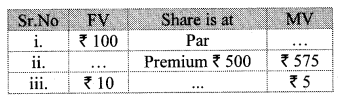Solution:
i. Here, share is at par.
∴ MV = FV
∴ MV = ₹ 100

ii. Here, Premium = ₹ 500, MV = ₹ 575
∴ FV + Premium = MV
∴ FV + 500 = 575
∴ FV = 575 – 500
∴ FV = ₹ 75

iii. Here, FV = ₹ 10, MV = ₹ 5
∴ FV > MV
Share is at discount.
FV – Discount = MV
∴ 10 – Discount = 5
∴ 10 – 5 = Discount
₹ Discount = ₹ 5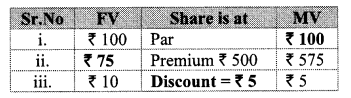Practice Set 4.3 Question 2. Mr. Amol purchased 50 shares of Face value ₹ 100 when the Market value of the share was ₹ 80. Company had given 20% dividend. Find the rate of return on investment.
Solution:
Here, MV = ₹ 80, FV = ₹ 100,
Number of shares = 50, Rate of dividend = 20%
∴ Sum invested = Number of shares × MV
= 50 × 80
= ₹ 4000

Dividend per share = 20% of FV
= $$\frac { 20 }{ 100 }$$ × 100 = ₹ 20
∴ Total dividend of 50 shares = 50 × 20
= ₹ 1000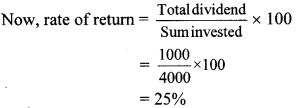∴ Rate of return on investment is 25%.

Practice Set 4.3 Question 3.
Joseph purchased following shares, Find his total investment.
Company A : 200 shares, FV = ₹ 2, Premium = ₹ 18.
Company B : 45 shares, MV = ₹ 500
Company C : 1 share, MV = ₹ 10,540
Solution:
For company A:
FV = ₹ 2, premium = ₹ 18,
Number of shares = 200
= 2 + 18
= ₹ 20
Sum invested = Number of shares × MV
= 200 × 20
= ₹ 14000

For company B:
MV = ₹ 500, Number of shares = 45
Sum invested = Number of shares × MV
= 45 × 500 = ₹ 22,500

For company C:
MV = ₹ 10,540, Number of shares = 1
∴ Sum invested = Number of shares × MV
= 1 × 10540
= ₹ 10,540
∴ Total investment of Joseph
= Investment for company A + Investment for company B + Investment for company C
= 4000 + 22,500 + 10,540
= ₹ 37040
∴ Total investment done by Joseph is ₹ 37,040.

Practice Set 4.3 Class 7th Question 4.
Smt. Deshpande purchased shares of FV ₹ 5 at a premium of ₹ 20. How many shares will she get for ₹ 20,000?
Solution:
Here, FV = ₹ 5, Premium = ₹ 20,
Sum invested = ₹ 20,000
∴ MV = FV + Premium
= 5 + 20
∴ MV = ₹ 25
Now, sum invested = Number of shares × MV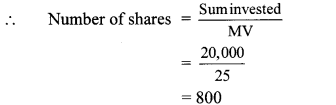∴ Smt. Deshpande got 800 shares for ₹ 20,000.

Question 5.
Shri Shantilal has purchased 150 shares of FV ₹ 100, for MV of ₹ 120. Company has paid dividend at 7%. Find the rate of return on his investment.
Solution:
Here, FV = ₹ 100, MV = ₹ 120
Dividend = 7%, Number of shares = 150
∴ Sum invested = Number of shares × MV
= 150 × 120 = ₹ 18000
Dividend per share = 7% of FV
= $$\frac { 7 }{ 100 }$$ × 100 = ₹ 7
∴ Total dividend of 150 shares
= 150 × 7 = ₹ 1050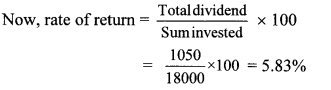∴ Rate of return on investment is 5.83%.

4.3 Class 10 Question 6. If the face value of both the shares is same, then which investment out of the following is more profitable?
Company A : dividend 16%, MV = ₹ 80,
Company B : dividend 20%, MV = ₹ 120.
Solution:
Let the face value of share be ₹ x.
For company A:
MV = ₹ 80, Dividend = 16%
Dividend = 16% of FV∴ Rate of return of company A is more.
∴ Investment in company A is profitable.

Question 1.
Smita has invested ₹ 12,000 and purchased shares of FV ₹ 10 at a premium of ₹ 2. Find the number of shares she purchased. Complete the given activity to get the answer. (Textbook pg. no. 101.)
Solution: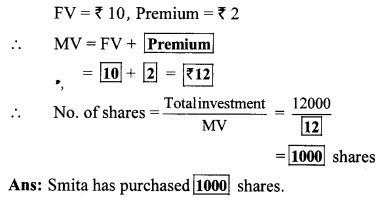Scroll to Top